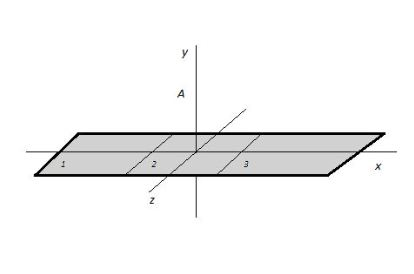# A strip of invisible tape 0.15 m long by 0.017 m wide is charged uniformly with a total net...

## Question:

A strip of invisible tape {eq}\displaystyle 0.15 m {/eq} long by {eq}\displaystyle 0.017 m {/eq} wide is charged uniformly with a total net charge of {eq}\displaystyle 4 \ nC {/eq} (nano = {eq}\displaystyle 1 \times 10^{9} {/eq}) and is suspended horizontally, so it lies along the x axis, with its center at the origin, as shown in the figure below. Calculate the approximate electric field at location {eq}\displaystyle \left \langle 0, 0.02, 0 \right \rangle m {/eq} (location A) due to the strip of tape. Do this by dividing the strip into three equal sections, as shown in the figure below, and approximating each section as a point charge. Assume each point charge is located at the center of the section it approximates. (Express answers in vector form)

(a) What is the approximate electric field at A due to piece 1?

(b) What is the approximate electric field at A due to piece 2?

(c) What is the approximate electric field at A due to piece 3?

(d) What is the approximate net electric field at A?## Electrostatic field

Coulomb's law states that the electrostatic force that appears between two point charges ({eq}q_1 {/eq} and {eq}q_2 {/eq}) is proportional to the product of their charges and inversely proportional to the square of the distance that separates ({eq}r {/eq}) them:

{eq}\displaystyle \vec{F}=\frac{kq_1q_2}{r^2}\hat{r}=q_1\vec{E}_2 {/eq}

where {eq}k=8.99\cdot10^9\,\mathrm{Nm^2/C^2} {/eq} is a constant, and {eq}\hat{r} {/eq} is a unit vector in the direction of the electric force, which can be attractive or repulsive.

In the other hand, according with Coulomb's law the electrostatic field generated by a point charge ({eq}q {/eq}) is determined by the expression:

{eq}\displaystyle \vec{E}=\frac{kq}{r^2}\hat{r} {/eq}

The point charges in each piece will be:

{eq}\displaystyle \sigma =\frac{Q_{net}}{A}\\ \displaystyle \sigma =\mathrm {\frac{4\cdot 10^{-9}}{(0.15\cdot 0.017)\,m^2}}\\ \displaystyle \sigma =\mathrm {1.6\cdot 10^{-6}\,C/m^2}\\ \displaystyle q_1=q_2=q_3=q=\sigma A_p\\ \displaystyle q=\mathrm {(1.6\cdot 10^{-6}\,C/m^2)(0.017\cdot 0.05\,m^2)}\\ \displaystyle q=\mathrm {1.4\cdot 10^{-9}\,C} {/eq}

a. The charge {eq}\displaystyle q_1 {/eq} is at a distance of {eq}\displaystyle \mathrm {-0.05\,m} {/eq} from the coordinate origin, on the x-axis. The electrostatic field due to this point charge would have one component x and another y given by:

{eq}\displaystyle \vec{E}_x=\frac{kq_1}{r^2}\cos \theta \hat{i}\\ \displaystyle \vec{E}_y=\frac{kq_1}{r^2}\sin \theta \hat{j} {/eq}

where {eq}\displaystyle \theta {/eq} is the angle formed between the unit vector {eq}\displaystyle \hat{r} {/eq} in the direction of the linear distance {eq}\displaystyle r {/eq} between the charge {eq}\displaystyle q_1 {/eq} and point {eq}\displaystyle A {/eq} to the point {eq}\displaystyle A {/eq} and the unit vector {eq}\displaystyle \hat{i} {/eq}.

{eq}\displaystyle r= \mathrm {\sqrt{(0.05\,m)^2+(0.02\,m)^2}}\\ \displaystyle r= \mathrm {0.054\,m}\\ \displaystyle \cos \theta =\mathrm {\frac{0.05\,m}{0.054\,m}}\\ \displaystyle \cos \theta =\mathrm {0.93}\\ \displaystyle \sin \theta =\mathrm {\frac{0.02\,m}{0.054\,m}}\\ \displaystyle \sin \theta =\mathrm {0.37} {/eq}

So the electrostatic field in {eq}\displaystyle A {/eq} because {eq}\displaystyle q_1 {/eq} is:

{eq}\displaystyle \vec{E}_1=\frac{kq_1}{r^2}\cos \theta \hat{i}+\frac{kq_1}{r^2}\sin \theta \hat{j}\\ \displaystyle \vec{E}_1=\mathrm {\frac{(8.99\cdot 10^9\,Nm^2/C^2)(1.4\cdot 10^{-9}\,C)}{0.03\,m^2}0.93 }\hat{i}+\mathrm {\frac{(8.99\cdot 10^9\,Nm^2/C^2)(1.4\cdot 10^{-9}\,C)}{0.03\,m^2}0.37}\hat{j}\\ \displaystyle \therefore \boxed{\vec{E}_1=(\mathrm {390}\hat{i}+\mathrm {144}\hat{j})\mathrm {\,N/C}} {/eq}

c. The charge {eq}\displaystyle q_1 {/eq} is at a distance of {eq}\displaystyle \mathrm {0.05\,m} {/eq} from the origin of the coordinates, on the x-axis. The electrostatic field in {eq}\displaystyle A {/eq} due to this point charge will be by symmetry equal to the field due to the charge {eq}\displaystyle q_1 {/eq}, only that its x-component will point in the opposite direction to that of {eq}\displaystyle q_1 {/eq}.

{eq}\displaystyle \therefore \boxed{\vec{E}_3=(\mathrm {-390}\hat{i}+\mathrm {144}\hat{j})\mathrm {\,N/C}} {/eq}

b. The charge {eq}\displaystyle q_2 {/eq} is at the origin of coordinates at a distance {eq}\displaystyle r_A {/eq} to the poit {eq}\displaystyle A {/eq}, so the electrostatic field in this point due to this charge will be:

{eq}\displaystyle \vec{E}_2=\frac{kq_1}{r^2_A}\hat{j}\\ \displaystyle \vec{E}_2=\mathrm {\frac{(8.99\cdot 10^9\,Nm^2/C^2)(1.4\cdot 10^{-9}\,C)}{(0.02\,m)^2}}\hat{j}\\ \displaystyle \therefore \boxed{\vec{E}_2=\mathrm {31465}\hat{j}\mathrm {\,N/C}} {/eq}

d. The net electrostatic field in {eq}\displaystyle A {/eq} will be the sum of the electrostatic fields due to the three point charges:

{eq}\displaystyle \therefore \boxed{\vec{E}_{net}=\mathrm {31853}\hat{j}\mathrm {\,N/C}} {/eq}